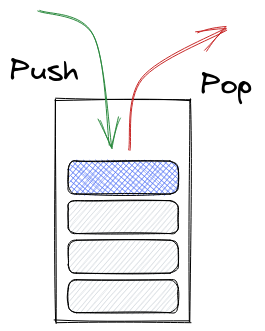# StackLanghjr265Replay of Cefalo SUST Int...
Limits 2s, 512 MB

StackLang is a stack-based programming language. Here, each program contains a series of commands that perform various stack operations.

A stack is a last-in-first-out data structure. You can push and pop elements to and from a stack. The element that has been pushed most recently is the top element. The element that comes out when a stack is popped is the element from the top.The stack used in StackLang can store 32-bit signed integers only and can store at most $10^6$ integers.

The language provides various commands to manipulate the stack:

• PUSH {V}: Push {V} into the stack. When the stack is full (i.e. it has $10^6$ elements), the bottom-most element is dropped.

• POP: Pop the top element from the stack. No change when the stack is empty.

• PRINT: Print the top element from the stack. Prints “-” when the stack is empty.

• SIZE: Print the number of elements in the stack. Prints “0” when the stack is empty.

• SUM: Print the summation of all the elements in the stack. Prints “0” when the stack is empty.

• REPEAT {N}: Repeats all the elements in the stack {N} times. If the stack currently has [1, 3, 7], 7 being at the top, then after executing the REPEAT 1 command, the stack will contain [1, 3, 7, 1, 3, 7]. When the stack is full, the bottom-most elements are dropped to make space for new elements from the repeat operation. Logically, a REPEAT N operation is like $N \times M$ PUSH commands, where $M$ is the number of elements in the stack immediately before the repeat operation begins.

• REVERSE: Reverses the entire stack. If the stack currently has [1, 3, 7], 7 being at the top, then after REVERSE, the stack will contain [7, 3, 1], 1 being at the top.

Here is an example StackLang program:

PUSH 1
PUSH 3
PUSH 7
PRINT
REVERSE
PRINT


This example StackLang program will output the following:

7
1


As you can see, in a well-formatted StackLang program, each command is in a separate line. There are no empty lines. For commands that accept a parameter, there is exactly one space between the command name and the parameter. The command names are always in uppercase.

In this problem, you have to implement an interpreter for StackLang. Easy, right?

## Input

All StackLang programs in the test cases will have at most $10^6$ lines of commands. Each command will be in a separate line. There will be no empty lines. You will have to read until EOF.

Your program should interpret the StackLang program provided in the input and perform actions against each command.

Commands PUSH and REPEAT take a single argument. There will be exactly one space between the command and the argument. All other commands do not take any argument.

In case of PUSH {V}, {V} will be a valid 32-bit signed integer.

In case of REPEAT {N}, {N} will be in the range $[1, 10^6]$ (between 1 and 1000000, inclusive).

## Output

You will print the output of PRINT, SIZE, and SUM commands, each in a separate line, as described in the problem statement.

## Samples

InputOutput
PUSH 1
PUSH 3
PUSH 7
PRINT
REVERSE
PRINT
SIZE
POP
SUM

7
1
3
10


After the first three operations you have [1, 3, 7] in the stack.

The command PRINT will then print 7 to the output, 7 being the element at the top of the stack. REVERSE will reverse the contents of the stack: [1, 3, 7] will become [7, 3, 1]. The next PRINT command will then print 1 to the output.

SIZE will print 3 to the output. POP will remove 1 from the stack. SUM will print the summation of 7 and 3: 10.

InputOutput
PUSH 1
PUSH 3
PUSH 7
REPEAT 1
PRINT
POP
PRINT
POP
PRINT
POP
PRINT
POP
PRINT

7
3
1
7
3### Submit